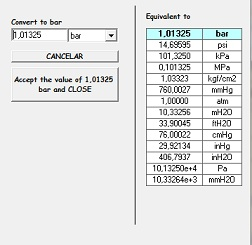# FlpAC - SOFTWARE FOR PRESSURE DROP CALCULATION IN PIPES AND FITTINGS

## Introduction

The purpose of FlpAC is the calculation of pressure losses for liquids and gases and to provide some other important parameters for the piping desgin:

• Reynolds number

• Volumetric and mass flow rates in any reference conditions. Correction for compressibility factor for gases.

• Density velocity and mass flow rate in actual process conditions.

• Friction factor and pressure drop (Colebrook / Darcy-Weisbach)

• Pressure drop in fittings.

•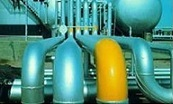Calculation of Reynolds number, friction factor and pressure drop in pipes, according to the Colebrook and Darcy-Weisbach equations.

The friction factor is a iterative and laborious calculation. FlpAC provides a simple and intuitive way to do it. It does not require a pre-established order for the input of data and so it can be used to solve different types of problems.

We need to enter only the minimum data necessary for the computation.

Friction factor can be calculated for both laminar and turbulent flow.

For Reynolds numbers between 2000 and 4000, the flow is within the critical zone, with some uncertainty about the flow behaviour. In this case the friction factor is estimated.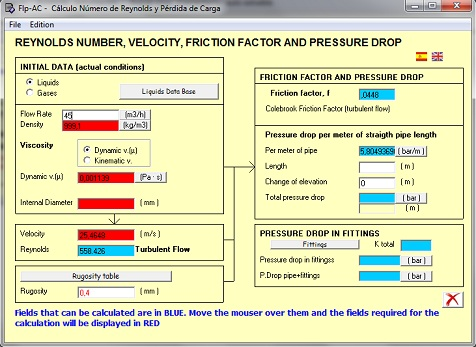#### Pressure drop in fittings

The application includes a data base with friction coefficients - k - . The friction coefficientes can also be entered manually.

#### Flow and density calculatin for gases. Referred to actual process conditions

The user interface is intutitive and the flow gas calculation can be expressed at any reference condition of pressure and temperature.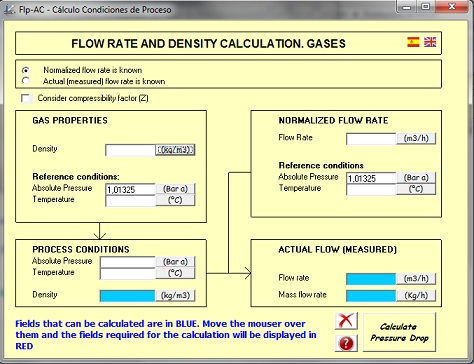#### Fitting selection

Data base with usual pipe fittings. K factor es determined as a function of type of fitting and size. The fittings can also be entered manually.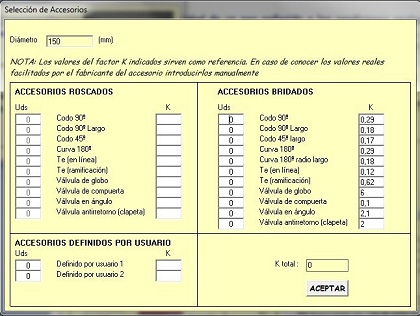.

#### Unit conversion

FlpAC software includes a built-in unit converter.